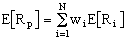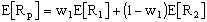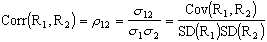Two Asset Portfolio Calculator
 Stock 1 Stock 2 Expected Return: % % Standard Deviation: % % Correlation Coefficient:
 Weight 1 Expected Return Variance Standard Deviation % % % % % % % % % % % % % % % % % % % % % % % % % % % % % % % % %

The Two Asset Portfolio Calculator can be used to find the Expected ReturnVariance, and Standard Deviation for portfolios formed from two assets.W1 and W2 are the percentage of each stock in the portfolio.• r12 = the correlation coefficient between the returns on stocks 1 and 2,
• s12 = the covariance between the returns on stocks 1 and 2,
• s1 = the standard deviation on stock 1, and
• s2 = the standard deviation on stock 2.
•• s12 = the covariance between the returns on stocks 1 and 2,
• N = the number of states,
• pi = the probability of state i,
• R1i = the return on stock 1 in state i,
• E[R1] = the expected return on stock 1,
• R2i = the return on stock 2 in state i, and
• E[R2] = the expected return on stock 2.
1. Expected Return Fields - Enter the Expected Return on Stocks 1 and 2 in these fields.
2. Standard Deviation Fields - Enter the Standard Deviation on Stocks 1 and 2 in these fields.
3. Correlation Coefficient Fields - Enter the Correlation Coefficient between the returns on Stocks 1 and 2 in this field.
4. Buttons - Press the Calculate button to calculate the Expected Return, Variance and Standard Deviation on portfolios formed from Stocks 1 and 2. Press the Clear button to clear the calculator.
5. Portfolio Returns - The Expected Return, Variance, and Standard Deviation for the portfolios formed from Stocks 1 and 2 are displayed in this table. For each row in the table, the first column indicates the weight of Stock 1 in the portfolio (the weight of Stock 2 is 100% minus the weight of Stock 1), the second column provides the Expected Return on the portfolio, the third column provides the Variance of the portfolio, and the fourth column provides the Standard Deviation of the portfolio.afds
copy right 2002 - 2019 by Mark A. Lane, Ph.D.